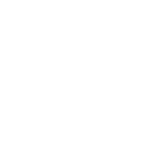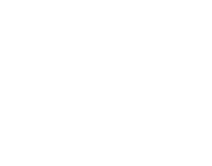# Volume and Capacity Questions and Answers - Form 1 Topical Mathematics

Questions

1. A village water tank is in the form of a frustrum of a cone of height 3.2m. The top and bottom radii are 18m and 24m respectively
1. Calculate:
1. The surface area of the tank excluding the bottom
2. The capacity of the water tank
2. 15 families each having 15 members use the water tank and each person uses 65 litres of water daily. How long will it take for the full tank to be emptied
2. The diagram below shows a bucket with a top diameter 30cm and bottom diameter 20cm. The height of the bucket is 28cm
1. Calculate the capacity of the bucket in litres2. Find the area of the metal sheet required to make 100 such buckets taking 10% extra for overlapping and wastage
3. A rectangular water tank measures 2.6m by 4.8m at the base and has water to a height of 3.2m. Find the volume of water in litres that is in the tank
4. The figure alongside shows a cone from which a frustum is made. A plane parallel to the base cuts the cone two thirds way up the vertical height of the cone to form frustum ABCDThe top surface radius of the frustum is labeled r and the bottom radius is R1. Find the ratio r:R
2. Given that r = 7cm, find R
3. If the height VY of the original cone is 45cm, calculate to the nearest whole number the volume of the frustum
4. The frustum represents a bucket which is used to fill a rectangular tank measuring 1.5m long, 1.2m wide and 80cm high with water. How many full buckets of water are required to fill the tank
5. Three litres of water (density1g/cm3) is added to twelve litres of alcohol (density 0.8g/cm3)What is the density of the mixture?
6.  A rectangular tank whose internal dimensions are 2.2m by 1.4m by 1.7m is three fifth full of milk.
1. Calculate the volume of milk in litres
2. The milk is packed in small packets in the shape of a right pyramid with an equilateral base triangle of sides 10cm. The vertical height of each packet is 13.6cm. Full packets obtained are sold at shs.30 per packet. Calculate:
1. The volume in cm3 of each packet to the nearest whole number
2. The number of full packets of milk
3. The amount of money realized from the sale of milk
7. An 890kg culvert is made of a hollow cylindrical material with outer radius of76cm and an inner radius of 64cm. It crosses a road of width 3m, determine the density of the material
used in its construction in Kg/m
3 correct to 1 decimal place.

1.  L.s.f. = 18/24 = 3/4
A.s.f = 9/16
v.s.f = 27/64
h/3.2 = 3/4 →4h = 3h + (3 x 3.2)
h = 9.6
1. surface area of small cone:
L = √(92 + 9.62) = 13.16m
S.A = (3.142 x 9 x 13.6) = 384.581
Curved area of frustrum = 7/1 x (3.142 x 9 x 13.16)/9
= 289.4
Top area = (3.142 x 92) = 254.5cm
Total area = 543.9m
2. Volume of smaller cone = (3.142 x 92 x 9.6)/3
= 814.41
Volume f frustrum = (37 x 814.41)/27
= 1116.043m3
= 1116043L
Litres used per day= (15 x 15 x 40) + (116 x 65)= 16540L
No. of days = 1116043/16540 = 67.5days
1. L.S.F = 3/2 = (28 + h)/h
56 + 2h = 3h
h = 56cm
Volume = 1/3 r2H – 1/3r2h
=1/3 x 22/7 x 15x 15x56 − 1/3 x 22/7 x 10 x 10 x 28
= 13200 – 29331/3
= 10.2667 litres
2. Slant height = 152 + 562 = 3361
= 57.97cm
Curved surface – RL – rl
2.  2.6 x 4.8 x 3.2 = 39.936m3
1m3 = 1000litres
39.936m3 = 39.936 x 1000
= 39936 litres
1. The top surface of the frustrum is −2/3 way up the vertical height of the original one.
VX: XY = 1/3h: h = 1:3
Using similar triangle we have
r/R = VX/VY = 1/3
r:R = 1:3
2. r/R = 1/3R = 3r
R = 3x7 = 21cm
3. height of removed cone is 1/3 height of original cone
h = 1/3 x 45 = 15cm
volume of removed cone = 1/3 r2h
= 1 x 22/7 x 7 x7 x15
= 770cm3
Now L. S. F = 1/3
V. S. F= (1/3)3 = 1/2
Hence ratio of volumes = 1:27
Volume of original cone = 27x Vol. of small cone
= 770 x 27= 20790cm3
Capacity of frustrum
= vol. of original cone – vol. of removed cone
= 20790 – 770= 20020cm3
20200/1000 = 20 l
4. capacity of tank = 150 x 120 x 80/1000 = 1440l
No. of buckets = 1440/20 = 72buckets
3. Mass of water = 1 x 3000 cm3 = 3000 g
Mass of alcohol = 0.8 x 1200 = 9600g
Mass of mixture = 12,600g
Volume of mixture = 15,000 cm3
Density of mixture = 12600/15000
= 0.84g/cm3
1. Vol. of tank = 22 x 144 x 1.7 = 5.236
Vol. of milk =
3/5 x 5.236 = 3.146m3
Vol. in liters = 3.1416 x 1000 = 3141.6litres
1. Vol. of packet (1/3 x 10 sin 60) x 13.6
= 26.97 x 13.6
= 3.66.75cm3
= 367cm3
2. No. packets = (3141.6 x 1000)/367
3. Amount = 8560.2 x 20
= 171204.3597
= Shs.171,204.40
4.  Volume of culvert
=22/7 (762 – 642) x 300 x 10-6
=22/7 x (1680 x 300)/10000000000
= 1.584m3

• ✔ To read offline at any time.
• ✔ To Print at your convenience
• ✔ Share Easily with Friends / Students